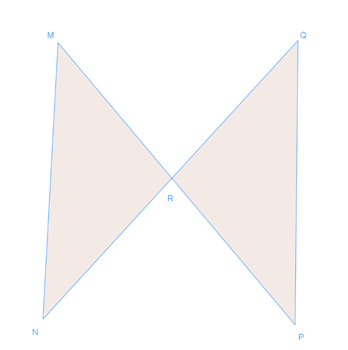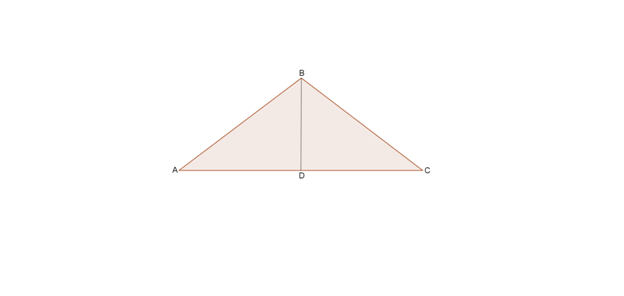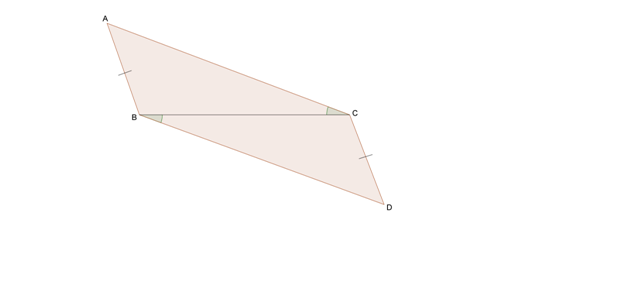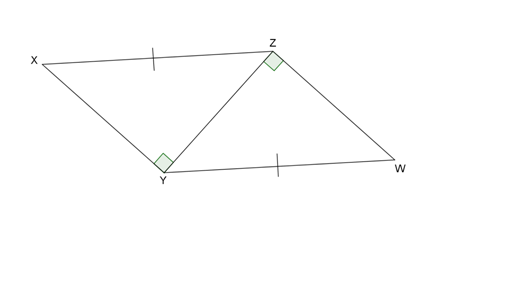# Triangles congruent by SAS and HL proofs#### All in One Place

Everything you need for JC, LC, and college level maths and science classes.#### Learn with Ease

We’ve mastered the national curriculum so that you can revise with confidence.#### Instant Help

0/1
##### Intros
###### Lessons
1. Similar tirangles VS. Congruent triangles
2. Ways to prove congruency:
• SSS
• SAS
• ASA
• AAS
0/6
##### Examples
###### Lessons
1. Write a two-column proof.
1. Given: R is the midpoint of $\overline {MP}$.
R is the midpoint of $\overline {NQ}$.
Prove: $\triangle MRN$$\cong$$\triangle PRQ$2. Special case for right triangle:
Given: $\triangle ABC$ is an isosceles triangle.
D is the midpoint of $\overline {AC}$.
Prove: $\triangle ABD$$\cong$$\triangle CBD$.2. Write a flow proof
1. Given: R is the midpoint of $\overline {MP}$.
R is the midpoint of $\overline {NQ}$.
Prove: $\triangle MRN$$\cong$$\triangle PRQ$2. Special case for right triangle:
Given: $\triangle ABC$ is an isosceles triangle.
D is the midpoint of $\overline {AC}$.
Prove: $\triangle ABD$$\cong$$\triangle CBD$.3. Is there enough information given to prove the following triangles are congruent? If so, explain your reasoning and point out the theorem or postulate you use.

1.2.